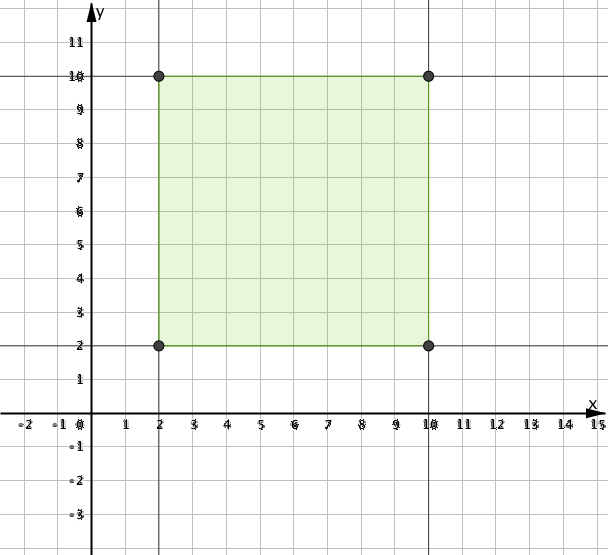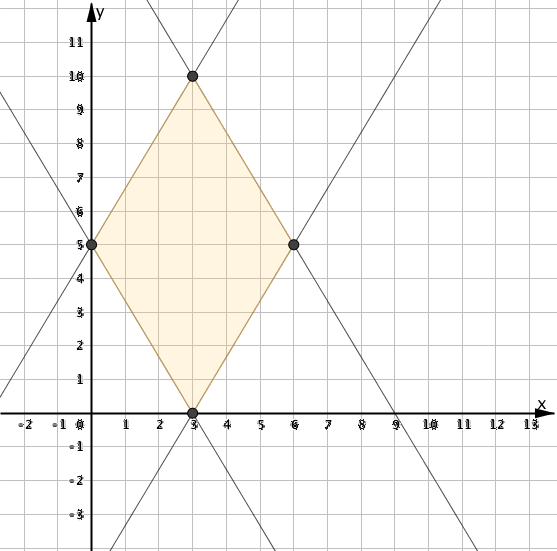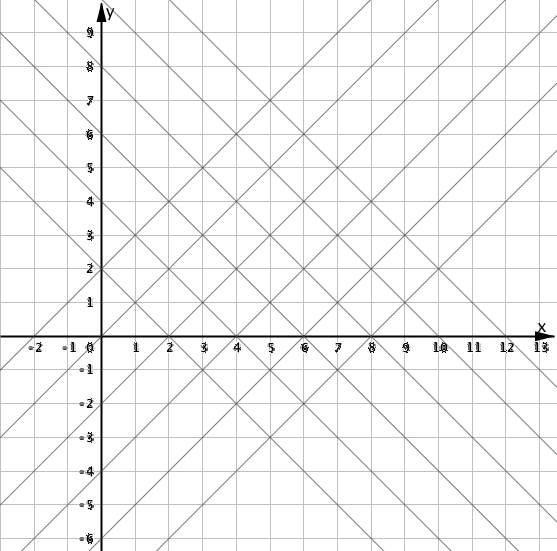# Linear Patterns

Check out patterns 1, 2, 3 and 4 (below). Use two points on each line to find the slope of each line. Then identify the vertical intercept if possible. Write the relation as an equation betweenand.

Plot the points and try out your equations here:

## Pattern 1ps – what’s the area of the quadrilateral bound by the four lines? Use the polygon tool to check out your answer.

## Pattern 2## Pattern 3## Pattern 4More Practice on Graphs of Linear Functions

Solutions More Practice on Drawing Graphs of Linear Functions Solutions